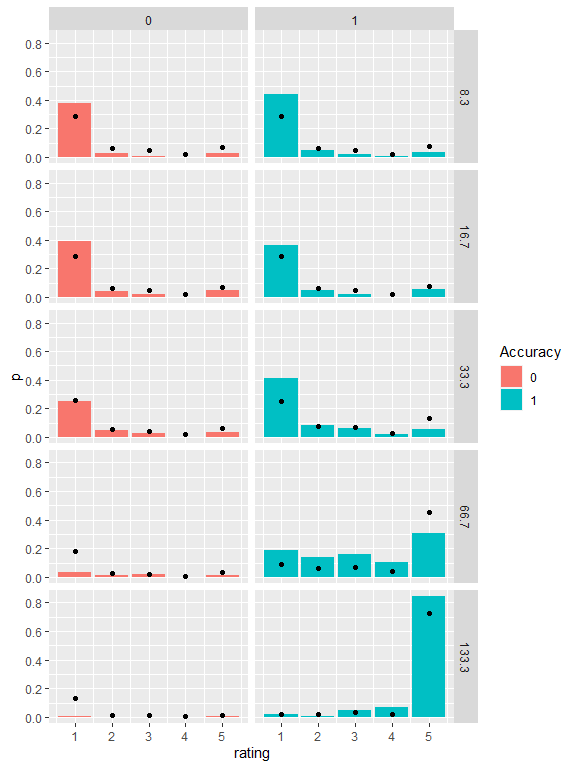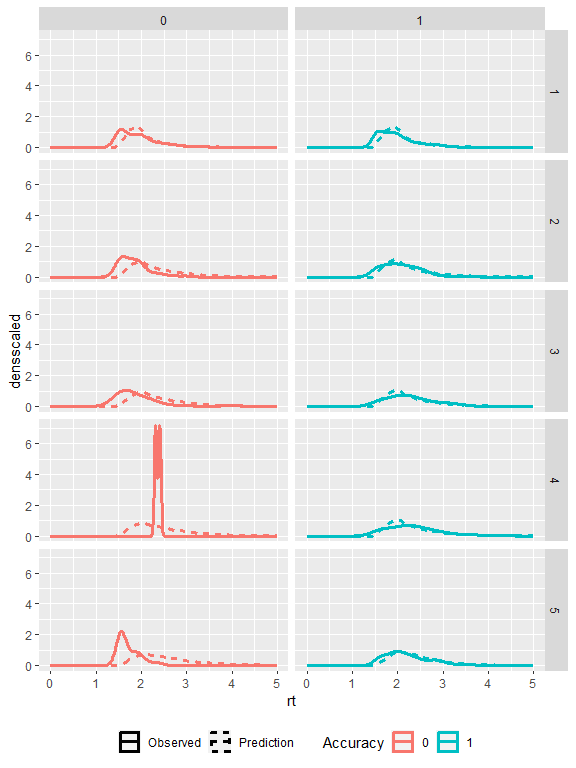# Fitting a dynamical confidence model to a data set

library(dynConfiR)
library(dplyr)
#>
#> Attache Paket: 'dplyr'
#> Die folgenden Objekte sind maskiert von 'package:stats':
#>
#>     filter, lag
#> Die folgenden Objekte sind maskiert von 'package:base':
#>
#>     intersect, setdiff, setequal, union
library(ggplot2)

This vignette shows how to fit the models and use model parameters to predict and simulate new data. It covers only how to get a quick fit to a single participant (i.e. only one set of fitted parameters) for a single model. The wrapper function fitRTConfModels may be used to fit several models to several independent participants in parallel and with one command, which usually takes quite a while (around several days depending also on the number of trials per participant).

The dynamic weighted evidence and visibility model (dynWEV) is one possible model. See https://osf.io/9jfqr/ for the theoretical description of this and the other models.

### Import Data

The ConfidenceOrientation dataset is included in the package and can be loaded after the package is loaded. We subset to work only with the data from one participant and pick only the theoretically relevant columns.

data("ConfidenceOrientation")
part8 <- ConfidenceOrientation %>%
filter(participant == 8) %>%
select(SOA, stimulus, response, rt, disc_rating)
#> # A tibble: 6 × 5
#>     SOA stimulus  response     rt disc_rating
#>   <dbl> <chr>     <chr>     <dbl>       <dbl>
#> 1  66.7 senkrecht senkrecht  2.45           5
#> 2   8.3 waagrecht senkrecht  2.63           1
#> 3   8.3 senkrecht senkrecht  3.16           1
#> 4  16.7 senkrecht senkrecht  2.61           1
#> 5   8.3 senkrecht senkrecht  3.83           1
#> 6 133.  senkrecht senkrecht  2.68           5

### Call fitting function

A specific model, in this example the dynWEV model, is fitted With a simple call to the function fitRTConf.

The fitting functions either require the columns condition, stimulus, response (or correct), rt, and rating to be present in the data argument or alternatively arguments that specify the mapping of column names to the required variables with e.g. condition="SOA". For simplicity we rename the column names before fitting, to have congruent column names in data and later predictions.

As the fitting procedure takes some time to deliver reasonable fits, we load previously fitted parameters into the environment (hidden code) and comment the actual function call.

To get a fast (but probably rather inaccurate) fit that is likely to represent only a locally minimizing maximum likelihood estimation one may use several strategies: - the argument grid_search=FALSE prevents the time consuming grid search for the best starting values - adjusting the values in the opts argument, which is a list, reduces the time of the actual optimization procedure - "nAttempts"=1 forces only the best set of initial parameters to be optimized - "nRestarts"=1 if one does not want to restart the optimization routine several times - one may also restrict some parameters that thus not have to be fit. Note, that this has theoretical implications on what the model may predict. There are several common restrictions - an unbiased starting point: z=0.5 - no variation in starting point: sz=0 - symmetric confidence thresholds for both possible responses: sym_thetas = TRUE - no variation in non-decision time: st0=0

Finally, as in this experiment confidence was reported simultaneously with the discrimination response, we set restr_tau= "simult_conf" (see documentation).

part8 <- part8 %>% rename(condition=SOA,
rating = disc_rating)
# parfit <- fitRTConf(part8, "dynWEV",
#                     restr_tau="simult_conf")
parfit
#>          v1         v2        v3        v4       v5        sv        a
#> 1 0.0372688 0.02975593 0.2286821 0.9073326 1.519281 0.7033667 1.990934
#>           z       sz         t0       st0 thetaLower1 thetaLower2 thetaLower3
#> 1 0.4840425 0.968085 0.01379258 0.5047345   0.9779324    1.400266    1.651361
#>   thetaLower4 thetaUpper1 thetaUpper2 thetaUpper3 thetaUpper4      tau        w
#> 1    1.826896   0.8949651    1.219724    1.674355    1.858899 1.497835 0.632632
#>         svis     sigvis             fixed negLogLik    N  k      BIC     AICc
#> 1 0.00228473 0.06989718 sym_thetas = TRUE  3130.487 1611 23 6430.819 6307.611
#>        AIC
#> 1 6306.973

### Prediction

After fitting the parameters to the empirical data, these parameters are used to compute the response and response time distribution predicted by the model. One could use the high-level functions predictConf and predictRT here (again for several participants and/or models there are the wrappers predictConfModels and predictRTModels), but we use predictWEV_Conf and predictWEV_Conf to specify the precision argument to speed up the computations.

predictedResponses <-
predictWEV_Conf(parfit, "dynWEV", simult_conf = TRUE,
precision = 1e-3, maxrt = 5, subdivisions = 50)
predictedRTdist <-
predictWEV_RT(parfit, "dynWEV", simult_conf = TRUE,
maxrt = 5, precision = 1e-3, subdivisions = 50,
scaled=TRUE, DistConf = predictedResponses)
#>   condition stimulus response correct rating          p info          err
#> 1         1        1        1       1      1 0.26584081   OK 6.893660e-07
#> 2         2        1        1       1      1 0.26705120   OK 6.916824e-07
#> 3         3        1        1       1      1 0.22905816   OK 6.192858e-07
#> 4         4        1        1       1      1 0.08011349   OK 2.837693e-07
#> 5         5        1        1       1      1 0.01564043   OK 1.173039e-04
#> 6         1       -1        1       0      1 0.26674388   OK 6.979109e-07
#>   condition stimulus response correct rating         rt dens densscaled
#> 1         1        1        1       1      1 0.01379258    0          0
#> 2         1        1        1       1      1 0.11555191    0          0
#> 3         1        1        1       1      1 0.21731125    0          0
#> 4         1        1        1       1      1 0.31907058    0          0
#> 5         1        1        1       1      1 0.42082992    0          0
#> 6         1        1        1       1      1 0.52258925    0          0

### Visual comparison

The predicted distributions may be visually compared to the empirical distributions to check how accurately the model fits the data. Therefore, we transform the condition column in the prediction data sets to fit the one in the empirical data and aggregate the data sets over stimulus and response identity and distinguish only correct and incorrect responses.

part8 <- part8 %>%
mutate(condition = as.factor(condition),
correct = as.numeric(stimulus==response))
empirical_response_dist <- part8 %>%
group_by(condition) %>%
mutate(ntrials = n()) %>%
group_by(correct, condition, rating) %>%
summarise(p = n()/ntrials, .groups = "drop")
predictedResponses <- predictedResponses %>%
mutate(condition = factor(condition, labels=levels(part8$condition))) %>% group_by(correct, condition, rating) %>% summarise(p = mean(p), .groups = "drop") predictedRTdist <- predictedRTdist %>% mutate(condition = factor(condition, labels=levels(part8$condition))) %>%
group_by(correct, rating, rt) %>%
summarise(dens = mean(dens),
densscaled = mean(densscaled), .groups = "drop")
ggplot(empirical_response_dist, aes(x=rating, y=p)) +
geom_bar(aes(fill=as.factor(correct)), stat="identity")+
geom_point(data=predictedResponses) +
scale_fill_discrete(name="Accuracy")+
facet_grid(cols=vars(correct), rows=vars(condition))ggplot(subset(part8, rt<18), aes(x=rt, color=as.factor(correct))) +
geom_density(aes(linetype="Observed"), size=1.2)+
geom_line(data = predictedRTdist,
aes(y=densscaled, linetype="Prediction"),
size=1.2)+
scale_color_discrete(name="Accuracy")+
scale_linetype_discrete(name="")+
theme(legend.position = "bottom")+
xlim(0, 5)+
facet_grid(rows=vars(rating), cols=vars(correct))
#> Warning: Using size aesthetic for lines was deprecated in ggplot2 3.4.0.
#> ℹ Please use linewidth instead.
#> This warning is displayed once every 8 hours.
#> Call lifecycle::last_lifecycle_warnings() to see where this warning was
#> generated.
#> Warning: Removed 4 rows containing non-finite values (stat_density()).